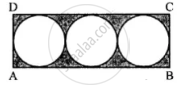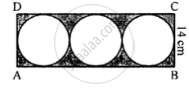# In the Following Figure, a Rectangle Abcd Enclosed Three Circles. If Bc = 14 Cm, Find the Area of the Shaded Portion (Take π = 22/7) - Mathematics

Sum

In the following figure, a rectangle ABCD enclosed three circles. If BC = 14 cm, find the area of the shaded portion (Take π = 22/7)#### Solution

In rectangle ABCD, BC = 14 cm∴ Radius of each circle = 14/2 = 7 cm

∴ Length of rectangle = (7 + 7 + 7) xx 2

= 42 cm

Now area of rectangle = l xx b = 42 xx 14

= 588 cm2

and area of 3 circles = 3 xx pir^2

= 3 xx 22/7 xx 7xx 7 = 462 cm2

= 588 - 462 = 126 cm2

Concept: Area of Circle
Is there an error in this question or solution?

#### APPEARS IN

Selina Concise Mathematics Class 8 ICSE
Chapter 20 Area of a Trapezium and a Polygon
Exercise 20 (D) | Q 18 | Page 235